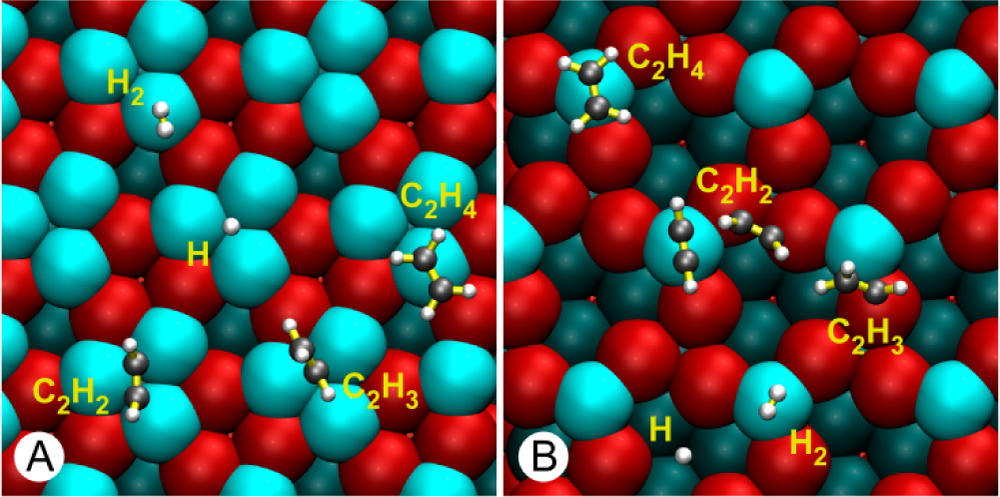# Adsorption of acetylene on an intermetallic surface

FILES CAN BE FOUND HERE: tar file

In this exercise you will compute the adsorption energy of acetylene on a intermetallic catalyst. This process is important during the production of polyethylene, and the system is described in this paper: 10.1021/ja505936b.

• In the first part of the exercise you will consider the optimized configuration (already in the directory) and study the pure electronic adsorption energy, namely the difference between the total energy of the surface-molecule system and the energy of the molecule alone and surface alone in the same geometry as the surface-molecule system minimum structure. This will allow to show the binding pattern of the electronic density.
• In the second part, you will optimize the surface and the molecule separately; this will allow to compute the total adsorption energy.The coordinates of the optimized configuration are provided to you as S_M.opt.xyz (S stands for “Substrate”, M for “Molecule”, opt for “optimized”). Visualize the geometry with VMD and familiarize yourself with the system.

## 2. Task: Bond induced density differences

Compute the density difference induced by the adsorption bonding. For this you will have to run three separate energy calculations, using the *.ene.inp files.

1. combined system (file S_M.opt.xyz)
2. lone acetylene (file M.S_M.xyz)
3. lone slab (file S.S_M.xyz)

In order to output the electronic densities as cube files, your input file has to contain the following snipped:

&DFT
&PRINT
&E_DENSITY_CUBE
&END E_DENSITY_CUBE
&END
&END DFT
The calculations involving the slab should be run with a reduced model with the serial cp2k cp2k.ssmp -i file.inp > file.out.

To process the cube files we are going to use the cubecruncher tool. It is part of CP2K and is in your exercise directory.

(aiida) max@qmobile:~/exercise_6$./cubecruncher.x -i S_M-ELECTRON_DENSITY-1_0.cube -subtract S.S_M-ELECTRON_DENSITY-1_0.cube -o tmp.cube (aiida) max@qmobile:~/exercise_6$ ./cubecruncher.x -i tmp.cube -subtract M.S_M-ELECTRON_DENSITY-1_0.cube -o Delta_ads.cube

The generated cube file is not aligned with the simulation cell. Center the cube file with the cubecruncher.x tool:

(aiida) max@qmobile:~/exercise_6\$ ./cubecruncher.x -center geo -i Delta_ads.cube -o Delta_ads-centered.cube

You can visualize the resulting file delta_ads-centered.cube with VMD. This has been covered in a previous exercise.

Compute the binding energy:

$E_\text{binding}=\sum E_\text{products} - \sum E_\text{reactants}$

For this you will need the energy values of three systems:

1. lone acetylene molecule (run geometry optimization, use energy of last step)
2. lone slab (you can use the already geometry optimized coordinates from S.opt.xyz at the end of the exercise)
You can not reuse the energy values for the lone sub-systems from the previous task. Since the unbound subsystems might relax into a different geometry, they have to be geometry optimized first.

## Questions

• Sketch briefly the geometry of the molecule when adsorbed and in the gas phase.
• Report the system energy for the bonded system, lone slab, and lone molecule.
• Can you estimate the contribution due to the geometry relaxation?
• Briefly report the bond induced density difference on the system.

## Required Files

When you are dealing with big systems and multiple atomic species, the input can be simplified by splitting it into multiple files. We are going to use separate files for the coordinates, the basis-sets, and the pseudo-potentials. All these files should reside in the same directory as the main input file.
The provided files are all in the directory of the exercises. Change the name of the xyz file accordingly in the input files.
S_M.ene.inp
&FORCE_EVAL
METHOD Quickstep
&DFT
&PRINT
&E_DENSITY_CUBE
&END E_DENSITY_CUBE
&END
BASIS_SET_FILE_NAME ./BR
POTENTIAL_FILE_NAME ./GR
&QS
EPS_DEFAULT 1.0E-10
METHOD GPW
EXTRAPOLATION ASPC
EXTRAPOLATION_ORDER 3
&END QS
&MGRID
CUTOFF 400
NGRIDS 5
&END
&SCF
MAX_SCF 20
SCF_GUESS RESTART
EPS_SCF 1.0E-5
&OT
PRECONDITIONER  FULL_SINGLE_INVERSE
MINIMIZER  CG
&END
&OUTER_SCF
MAX_SCF 50
EPS_SCF 1.0E-5
&END
&PRINT
&END
&END SCF
&XC
&XC_FUNCTIONAL PBE
&END XC_FUNCTIONAL
&END XC
&POISSON
PERIODIC XY
POISSON_SOLVER ANALYTIC
&END
&END DFT
&SUBSYS
&CELL
A [angstrom] 7.042785 0 0
B [angstrom] 0  6.09925  0.
C [angstrom] 0.000000   0.   15.
PERIODIC XY
&END CELL
&TOPOLOGY
COORD_FILE_NAME ./S_M.opt.xyz
COORDINATE xyz
&END
&KIND Pd
BASIS_SET DZVP-MOLOPT-SR-GTH-q18
POTENTIAL GTH-PBE-q18
&END KIND
&KIND Ga
BASIS_SET DZVP-MOLOPT-SR-GTH-q13
POTENTIAL GTH-PBE-q13
&END KIND
&KIND C
BASIS_SET TZV2P-MOLOPT-GTH
POTENTIAL GTH-PBE-q4
&END KIND
&KIND H
BASIS_SET TZV2P-MOLOPT-GTH
POTENTIAL GTH-PBE-q1
&END KIND
&END SUBSYS
&END FORCE_EVAL
&GLOBAL
PRINT_LEVEL LOW
PROJECT S_M
RUN_TYPE ENERGY
WALLTIME 860000
&END GLOBAL
&END


<